Setup for 4 Player a Game of Food Chain Magnate

Originally generated on 1/11/2019 11:16:40 AM

Player Setup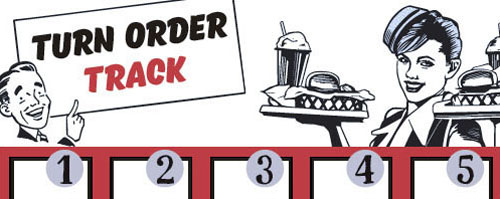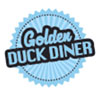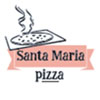Other Setup

Bank starts with \$200.00
Remove these Billboards: #16
Number of 1x Employee cards used = 2

Map (The Way Out Suburbs)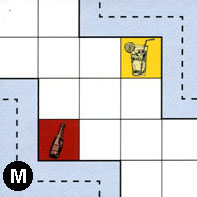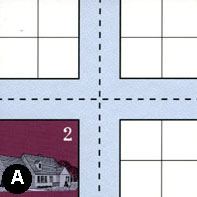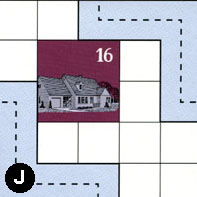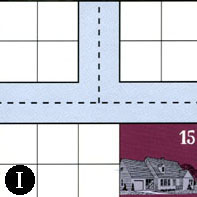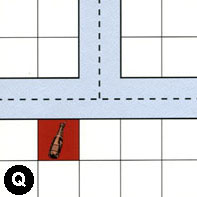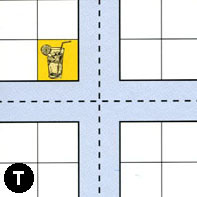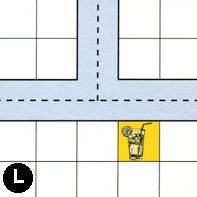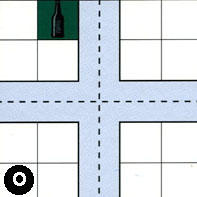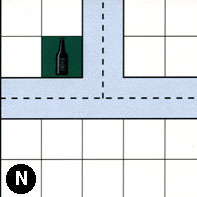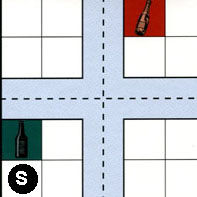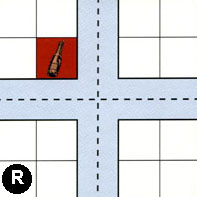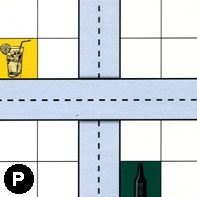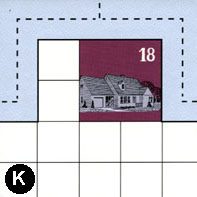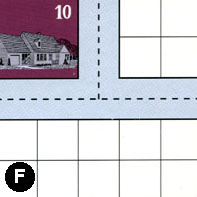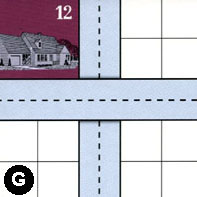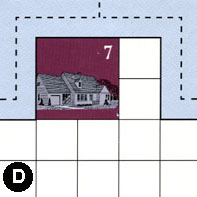Map Stats

Map Option = The Way Out Suburbs
Total Number of Tiles = 16
Total Number of Starting Houses = 7
Total Number of Beer Spots = 4
Total Number of Soda Spots = 4
Total Number of Lemonade Spots = 4
Total Number of Drink Spots = 12
Number of independent path systems = 4
Number of independent neighborhoods = 14

Path System 1
Size = Medium
Number of Tiles = 11
Number of Paths = 38
Contains Loop(s) = No
Number of Starting Houses = 4
Starting Houses Pct of Map (%) = 57
Number of Beer Spots = 2
Number of Soda Spots = 4
Number of Lemonade Spots = 4
Number of Drink Spots = 10
Drink Spots Pct of Map (%) = 83
Tile Ids = A, G, J, L, M, N, P, Q, R, S, T
Paths = MWS, AWN, ANE, JWS, LWE, RWN, SWE, NWN, QWN, QNE, MEN, QWE, NWE, NNE, SWN, TWN, TNE, AWS, AWE, AES, TWE, SNE, RWE, PWE, RNE, LWN, TWS, SNS, SWS, SES, RWS, GWE, RNS, RES, TNS, TES, ANS, LNE What do these mean?
Starting House Ids = 0, 2, 12, 16
Path System 2
Size = Small
Number of Tiles = 2
Number of Paths = 4
Contains Loop(s) = No
Number of Starting Houses = 2
Starting Houses Pct of Map (%) = 29
Number of Beer Spots = 0
Number of Soda Spots = 0
Number of Lemonade Spots = 0
Number of Drink Spots = 0
Drink Spots Pct of Map (%) = 0
Tile Ids = I, J
Paths = JEN, IWN, INE, IWE What do these mean?
Starting House Ids = 15, 16
Path System 3
Size = Medium
Number of Tiles = 2
Number of Paths = 7
Contains Loop(s) = No
Number of Starting Houses = 1
Starting Houses Pct of Map (%) = 14
Number of Beer Spots = 2
Number of Soda Spots = 0
Number of Lemonade Spots = 0
Number of Drink Spots = 2
Drink Spots Pct of Map (%) = 17
Tile Ids = O, P
Paths = OWS, PNS, OWN, ONE, OWE, OES, ONS What do these mean?
Starting House Ids = 0
Path System 4
Size = Medium
Number of Tiles = 4
Number of Paths = 10
Contains Loop(s) = No
Number of Starting Houses = 4
Starting Houses Pct of Map (%) = 57
Number of Beer Spots = 0
Number of Soda Spots = 0
Number of Lemonade Spots = 0
Number of Drink Spots = 0
Drink Spots Pct of Map (%) = 0
Tile Ids = D, F, G, K
Paths = KWN, FWE, GNS, DWE, DWN, DNE, FWN, FNE, KWE, KNE What do these mean?
Starting House Ids = 7, 10, 12, 18

Neighborhood 1
Total Size = Large
Number of Total Spaces = 45
Number of Empty Spaces (for new houses & gardens) = 36
Number of Beer Spots = 1
Number of Soda Spots = 2
Number of Lemonade Spots = 2
Number of Drink Spots = 5
Number of Starting Houses = 1
Starting Houses Pct of Map (%) = 14
Tile Ids = A, M, N, Q, S, T
Starting House Ids = 2
Neighborhood 2
Total Size = Small
Number of Total Spaces = 4
Number of Empty Spaces (for new houses & gardens) = 4
Number of Beer Spots = 0
Number of Soda Spots = 0
Number of Lemonade Spots = 0
Number of Drink Spots = 0
Number of Starting Houses = 0
Starting Houses Pct of Map (%) = 0
Tile Ids = A
Neighborhood 3
Total Size = Large
Number of Total Spaces = 59
Number of Empty Spaces (for new houses & gardens) = 48
Number of Beer Spots = 2
Number of Soda Spots = 0
Number of Lemonade Spots = 1
Number of Drink Spots = 3
Number of Starting Houses = 2
Starting Houses Pct of Map (%) = 29
Tile Ids = A, I, J, L, O, P, R
Starting House Ids = 15, 16
Neighborhood 4
Total Size = Small
Number of Total Spaces = 4
Number of Empty Spaces (for new houses & gardens) = 4
Number of Beer Spots = 0
Number of Soda Spots = 0
Number of Lemonade Spots = 0
Number of Drink Spots = 0
Number of Starting Houses = 0
Starting Houses Pct of Map (%) = 0
Tile Ids = I
Neighborhood 5
Total Size = Small
Number of Total Spaces = 4
Number of Empty Spaces (for new houses & gardens) = 4
Number of Beer Spots = 0
Number of Soda Spots = 0
Number of Lemonade Spots = 0
Number of Drink Spots = 0
Number of Starting Houses = 0
Starting Houses Pct of Map (%) = 0
Tile Ids = I
Neighborhood 6
Total Size = Medium
Number of Total Spaces = 12
Number of Empty Spaces (for new houses & gardens) = 12
Number of Beer Spots = 0
Number of Soda Spots = 0
Number of Lemonade Spots = 0
Number of Drink Spots = 0
Number of Starting Houses = 0
Starting Houses Pct of Map (%) = 0
Tile Ids = A, L, T
Neighborhood 7
Total Size = Small
Number of Total Spaces = 4
Number of Empty Spaces (for new houses & gardens) = 4
Number of Beer Spots = 0
Number of Soda Spots = 0
Number of Lemonade Spots = 0
Number of Drink Spots = 0
Number of Starting Houses = 0
Starting Houses Pct of Map (%) = 0
Tile Ids = Q
Neighborhood 8
Total Size = Large
Number of Total Spaces = 60
Number of Empty Spaces (for new houses & gardens) = 55
Number of Beer Spots = 1
Number of Soda Spots = 0
Number of Lemonade Spots = 0
Number of Drink Spots = 1
Number of Starting Houses = 1
Starting Houses Pct of Map (%) = 14
Tile Ids = F, G, K, N, Q, R, S
Starting House Ids = 18
Neighborhood 9
Total Size = Medium
Number of Total Spaces = 16
Number of Empty Spaces (for new houses & gardens) = 14
Number of Beer Spots = 0
Number of Soda Spots = 2
Number of Lemonade Spots = 0
Number of Drink Spots = 2
Number of Starting Houses = 0
Starting Houses Pct of Map (%) = 0
Tile Ids = L, R, S, T
Neighborhood 10
Total Size = Medium
Number of Total Spaces = 8
Number of Empty Spaces (for new houses & gardens) = 8
Number of Beer Spots = 0
Number of Soda Spots = 0
Number of Lemonade Spots = 0
Number of Drink Spots = 0
Number of Starting Houses = 0
Starting Houses Pct of Map (%) = 0
Tile Ids = O, P
Neighborhood 11
Total Size = Large
Number of Total Spaces = 32
Number of Empty Spaces (for new houses & gardens) = 27
Number of Beer Spots = 0
Number of Soda Spots = 0
Number of Lemonade Spots = 1
Number of Drink Spots = 1
Number of Starting Houses = 1
Starting Houses Pct of Map (%) = 14
Tile Ids = D, G, P, R
Starting House Ids = 7
Neighborhood 12
Total Size = Small
Number of Total Spaces = 4
Number of Empty Spaces (for new houses & gardens) = 4
Number of Beer Spots = 0
Number of Soda Spots = 0
Number of Lemonade Spots = 0
Number of Drink Spots = 0
Number of Starting Houses = 0
Starting Houses Pct of Map (%) = 0
Tile Ids = F
Neighborhood 13
Total Size = Medium
Number of Total Spaces = 8
Number of Empty Spaces (for new houses & gardens) = 0
Number of Beer Spots = 0
Number of Soda Spots = 0
Number of Lemonade Spots = 0
Number of Drink Spots = 0
Number of Starting Houses = 2
Starting Houses Pct of Map (%) = 29
Tile Ids = F, G
Starting House Ids = 10, 12
Neighborhood 14
Total Size = Small
Number of Total Spaces = 4
Number of Empty Spaces (for new houses & gardens) = 4
Number of Beer Spots = 0
Number of Soda Spots = 0
Number of Lemonade Spots = 0
Number of Drink Spots = 0
Number of Starting Houses = 0
Starting Houses Pct of Map (%) = 0
Tile Ids = G

v2.1.5